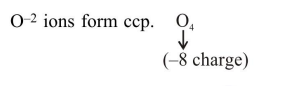# A crystal is made up of metal ions 'M1' and 'M2' and oxide ions.

Question:

A crystal is made up of metal ions ' $M_{1}{ }^{\prime}$ ana ' $M_{2}{ }^{\prime}$ and oxide ions. Oxide ions form a ccp lattice structure. The cation ' $\mathrm{M}_{1}{ }^{\prime}$ occupies $50 \%$ of octahedral voids and the cation ' $M_{2}$ ' occupies $12.5 \%$ of tetrahedral voids of oxide lattice. The oxidation numbers of ' $\mathrm{M}_{1}$ ' and ' $\mathrm{M}_{2}$ ' are, respectively:

1. $+2,+4$

2. $+3,+1$

3.  $+1,+3$

4. $+4,+2$

Correct Option: 1

Solution:$\mathrm{M}_{1}=50 \%$ of O.V. $\Rightarrow \frac{50}{100} \times 4=2:\left(\mathrm{M}_{1}\right)_{2}$

$\mathrm{M}_{2}=12.5 \%$ of T.V. $\Rightarrow \frac{12.5}{100} \times 8=1:\left(\mathrm{M}_{2}\right)_{1}$

So formula is : $\left(\mathrm{M}_{1}\right)_{2}\left(\mathrm{M}_{2}\right)_{1} \mathrm{O}_{4}$

This must be neutral. Both metals must have $+8$ charge in total.

From given options : $\left\{\begin{array}{r}\text { O.N. of } M_{1}=+2 \\ M_{2}=+4\end{array}\right\}$